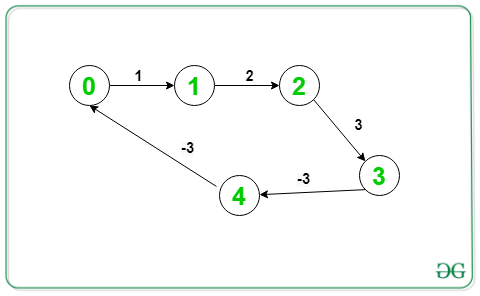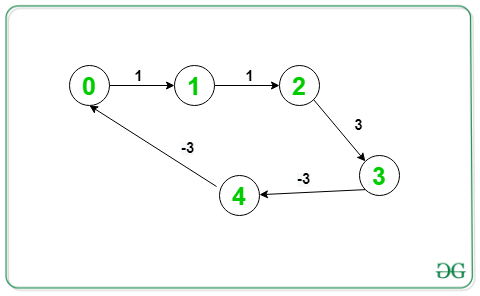Open In App

# Print negative weight cycle in a Directed Graph

Given a weighted directed graph consisting of V vertices and E edges. The task is to print the cyclic path whose sum of weight is negative. If there is no such path present then print “-1”

Input: V = 5, E = 5, Below is the graph:

Here, for the given negative cycle o/p (1->2->3->4->1) ; In fig there has to be Edge from 4–>1  not from 4–>0Output: 1 2 3 4 1
Explanation:
Given graph contains a negative cycle, (1->2->3->4->1)

Input: V = 5, E = 5, Below is the graph:Output: 0 1 2 3 4 0
Explanation:
Given graph contains a negative cycle, (0->1->2->3->4->0)

Approach: The idea is to use Bellman-Ford Algorithm which is used to detect a negative cycle or not. To print the negative cycles, perform the Nth iteration of Bellman-Ford and pick a vertex from any edge which is relaxed in this iteration. Using this vertex and its ancestors, the negative cycle can be printed. Below are the steps:

• Perform N-1 iterations of Bellman-Ford algorithm and relax each edge (u, v). Keep track of parent of each vertex and store in an array parent[].
• Now, do one more iteration and if no edge relaxation take place in this Nth iteration, then there is no cycle of negative weight exists in the graph.
• Otherwise take a variable C and store the vertex v from any edge (u, v), which is relaxed in the Nth iteration.
• Now, starting from the C vertex go towards its ancestors until a cycle is found and finally print it.
• This cycle will be the desired cycle of negative weight.

Below is the implementation of the above approach:

## C++

 `// C++ program for the above approach``#include ``using` `namespace` `std;` `// Structure to represent a weighted``// edge in graph``struct` `Edge {``    ``int` `src, dest, weight;``};` `// Structure to represent a directed``// and weighted graph``struct` `Graph {` `    ``// V -> Number of vertices,``    ``// E -> Number of edges``    ``int` `V, E;` `    ``// Graph is represented as an``    ``// array of edges``    ``struct` `Edge* edge;``};` `// Creates a new graph with V vertices``// and E edges``struct` `Graph* createGraph(``int` `V, ``int` `E)``{``    ``struct` `Graph* graph = ``new` `Graph;``    ``graph->V = V;``    ``graph->E = E;``    ``graph->edge = ``new` `Edge[graph->E];``    ``return` `graph;``}` `vector<``int``> vis;``// Function runs Bellman-Ford algorithm``// and prints negative cycle(if present)``void` `NegCycleBellmanFord(``struct` `Graph* graph,``                        ``int` `src)``{``    ``int` `V = graph->V;``    ``int` `E = graph->E;``    ``int` `dist[V];``    ``int` `parent[V];` `    ``// Initialize distances from src``    ``// to all other vertices as INFINITE``    ``// and all parent as -1``    ``for` `(``int` `i = 0; i < V; i++) {` `        ``dist[i] = INT_MAX;``        ``parent[i] = -1;``    ``}``    ``dist[src] = 0;``    ``vis[src] = 0;``    ``// Relax all edges |V| - 1 times.``    ``bool` `flg = ``true``;``    ``for` `(``int` `i = 1; i <= V - 1; i++) {``      ``if``(flg==``false``)``            ``break``;``      ``flg=``false``;``        ``for` `(``int` `j = 0; j < E; j++) {` `            ``int` `u = graph->edge[j].src;``            ``int` `v = graph->edge[j].dest;``            ``int` `weight = graph->edge[j].weight;` `            ``if` `(dist[u] != INT_MAX``                ``&& dist[u] + weight < dist[v]) {``                ``flg = ``true``;``                  ``vis[v] = 1;``                ``dist[v] = dist[u] + weight;``                ``parent[v] = u;``            ``}``        ``}``    ``}` `    ``// Check for negative-weight cycles``    ``int` `C = -1;``    ``for` `(``int` `i = 0; i < E; i++) {` `        ``int` `u = graph->edge[i].src;``        ``int` `v = graph->edge[i].dest;``        ``int` `weight = graph->edge[i].weight;` `        ``if` `(dist[u] != INT_MAX``            ``&& dist[u] + weight < dist[v]) {` `            ``// Store one of the vertex of``            ``// the negative weight cycle``            ``C = v;``            ``break``;``        ``}``    ``}` `    ``if` `(C != -1) {` `        ``for` `(``int` `i = 0; i < V; i++)``            ``C = parent[C];` `        ``// To store the cycle vertex``        ``vector<``int``> cycle;``        ``for` `(``int` `v = C;; v = parent[v]) {` `            ``cycle.push_back(v);``            ``if` `(v == C``                ``&& cycle.size() > 1)``                ``break``;``        ``}` `        ``// Reverse cycle[]``        ``reverse(cycle.begin(), cycle.end());` `        ``// Printing the negative cycle``        ``for` `(``int` `v : cycle)``            ``cout << v << ``' '``;``        ``cout << endl;``       ``return``;``    ``}``}` `// Driver Code``int` `main()``{``    ``// Number of vertices in graph``    ``int` `V = 5;` `    ``// Number of edges in graph``    ``int` `E = 5;` `    ``struct` `Graph* graph = createGraph(V, E);``    ``vis.resize(V,0);``    ``// Given Graph``    ``graph->edge.src = 1;``    ``graph->edge.dest = 0;``    ``graph->edge.weight = 1;` `    ``graph->edge.src = 1;``    ``graph->edge.dest = 2;``    ``graph->edge.weight = 2;` `    ``graph->edge.src = 2;``    ``graph->edge.dest = 3;``    ``graph->edge.weight = 3;` `    ``graph->edge.src = 3;``    ``graph->edge.dest = 4;``    ``graph->edge.weight = -3;` `    ``graph->edge.src = 4;``    ``graph->edge.dest = 1;``    ``graph->edge.weight = -3;` `    ``graph->edge.src = 5;``    ``graph->edge.dest = 6;``    ``graph->edge.weight = -1;` `    ``graph->edge.src = 6;``    ``graph->edge.dest = 7;``    ``graph->edge.weight =-1;` `    ``graph->edge.src = 7;``    ``graph->edge.dest = 5;``    ``graph->edge.weight =-1;``  ` `    ``// Function Call``      ``for``(``int` `src = 0;src

## Java

 `// Java program for the above approach``import` `java.util.ArrayList;``import` `java.util.Collections;` `class` `GFG{` `// Structure to represent a weighted``// edge in graph``static` `class` `Edge``{``    ``int` `src, dest, weight;``}` `// Structure to represent a directed``// and weighted graph``static` `class` `Graph``{``    ` `    ``// V. Number of vertices, E.``    ``// Number of edges``    ``int` `V, E;` `    ``// Graph is represented as``    ``// an array of edges.``    ``Edge[] edge;``}` `// Creates a new graph with V vertices``// and E edges``static` `Graph createGraph(``int` `V, ``int` `E)``{``    ``Graph graph = ``new` `Graph();``    ``graph.V = V;``    ``graph.E = E;``    ``graph.edge = ``new` `Edge[graph.E];` `    ``for``(``int` `i = ``0``; i < graph.E; i++)``    ``{``        ``graph.edge[i] = ``new` `Edge();``    ``}` `    ``return` `graph;``}` `// Function runs Bellman-Ford algorithm``// and prints negative cycle(if present)``static` `void` `NegCycleBellmanFord(Graph graph, ``int` `src)``{``    ``int` `V = graph.V;``    ``int` `E = graph.E;``    ``int``[] dist = ``new` `int``[V];``    ``int``[] parent = ``new` `int``[V];` `    ``// Initialize distances from src``    ``// to all other vertices as INFINITE``    ``// and all parent as -1``    ``for``(``int` `i = ``0``; i < V; i++)``    ``{``        ``dist[i] = ``1000000``;``        ``parent[i] = -``1``;``    ``}``    ``dist[src] = ``0``;` `    ``// Relax all edges |V| - 1 times.``    ``for``(``int` `i = ``1``; i <= V - ``1``; i++)``    ``{``        ``for``(``int` `j = ``0``; j < E; j++)``        ``{``            ``int` `u = graph.edge[j].src;``            ``int` `v = graph.edge[j].dest;``            ``int` `weight = graph.edge[j].weight;` `            ``if` `(dist[u] != ``1000000` `&&``                ``dist[u] + weight < dist[v])``            ``{``                ``dist[v] = dist[u] + weight;``                ``parent[v] = u;``            ``}``        ``}``    ``}` `    ``// Check for negative-weight cycles``    ``int` `C = -``1``;``    ``for``(``int` `i = ``0``; i < E; i++)``    ``{``        ``int` `u = graph.edge[i].src;``        ``int` `v = graph.edge[i].dest;``        ``int` `weight = graph.edge[i].weight;` `        ``if` `(dist[u] != ``1000000` `&&``            ``dist[u] + weight < dist[v])``        ``{``            ` `            ``// Store one of the vertex of``            ``// the negative weight cycle``            ``C = v;``            ``break``;``        ``}``    ``}` `    ``if` `(C != -``1``)``    ``{``        ``for``(``int` `i = ``0``; i < V; i++)``            ``C = parent[C];` `        ``// To store the cycle vertex``        ``ArrayList cycle = ``new` `ArrayList<>();``        ``for``(``int` `v = C;; v = parent[v])``        ``{``            ``cycle.add(v);``            ` `            ``if` `(v == C && cycle.size() > ``1``)``                ``break``;``        ``}` `        ``// Reverse cycle[]``        ``Collections.reverse(cycle);` `        ``// Printing the negative cycle``        ``for``(``int` `v : cycle)``            ``System.out.print(v + ``" "``);``            ` `        ``System.out.println();``    ``}``    ``else``        ``System.out.println(-``1``);``}` `// Driver Code``public` `static` `void` `main(String[] args)``{``    ` `    ``// Number of vertices in graph``    ``int` `V = ``5``;` `    ``// Number of edges in graph``    ``int` `E = ``5``;` `    ``Graph graph = createGraph(V, E);` `    ``// Given Graph``    ``graph.edge[``0``].src = ``0``;``    ``graph.edge[``0``].dest = ``1``;``    ``graph.edge[``0``].weight = ``1``;` `    ``graph.edge[``1``].src = ``1``;``    ``graph.edge[``1``].dest = ``2``;``    ``graph.edge[``1``].weight = ``2``;` `    ``graph.edge[``2``].src = ``2``;``    ``graph.edge[``2``].dest = ``3``;``    ``graph.edge[``2``].weight = ``3``;` `    ``graph.edge[``3``].src = ``3``;``    ``graph.edge[``3``].dest = ``4``;``    ``graph.edge[``3``].weight = -``3``;` `    ``graph.edge[``4``].src = ``4``;``    ``graph.edge[``4``].dest = ``1``;``    ``graph.edge[``4``].weight = -``3``;` `    ``// Function Call``    ``NegCycleBellmanFord(graph, ``0``);``}``}` `// This code is contributed by sanjeev2552`

## Python3

 `# Python3 program for the above approach`` ` `# Structure to represent a weighted``# edge in graph``class` `Edge:  ``    ``def` `__init__(``self``):``        ``self``.src ``=` `0``        ``self``.dest ``=` `0``        ``self``.weight ``=` `0` `# Structure to represent a directed``# and weighted graph``class` `Graph:` `    ``def` `__init__(``self``):``        ` `        ``# V. Number of vertices, E.``        ``# Number of edges``        ``self``.V ``=` `0``        ``self``.E ``=` `0``        ` `        ``# Graph is represented as``        ``# an array of edges.``        ``self``.edge ``=` `[]``     ` `# Creates a new graph with V vertices``# and E edges``def` `createGraph(V, E):``    ``graph ``=` `Graph();``    ``graph.V ``=` `V;``    ``graph.E ``=` `E;``    ``graph.edge ``=` `[Edge() ``for` `i ``in` `range``(graph.E)]``    ``return` `graph;``  ` `# Function runs Bellman-Ford algorithm``# and prints negative cycle(if present)``def` `NegCycleBellmanFord(graph, src):``    ``V ``=` `graph.V;``    ``E ``=` `graph.E;``    ``dist ``=``[``1000000` `for` `i ``in` `range``(V)]``    ``parent ``=``[``-``1` `for` `i ``in` `range``(V)]``    ``dist[src] ``=` `0``;`` ` `    ``# Relax all edges |V| - 1 times.``    ``for` `i ``in` `range``(``1``, V):``        ``for` `j ``in` `range``(E):``    ` `            ``u ``=` `graph.edge[j].src;``            ``v ``=` `graph.edge[j].dest;``            ``weight ``=` `graph.edge[j].weight;`` ` `            ``if` `(dist[u] !``=` `1000000` `and``                ``dist[u] ``+` `weight < dist[v]):``            ` `                ``dist[v] ``=` `dist[u] ``+` `weight;``                ``parent[v] ``=` `u;`` ` `    ``# Check for negative-weight cycles``    ``C ``=` `-``1``;   ``    ``for` `i ``in` `range``(E):  ``        ``u ``=` `graph.edge[i].src;``        ``v ``=` `graph.edge[i].dest;``        ``weight ``=` `graph.edge[i].weight;`` ` `        ``if` `(dist[u] !``=` `1000000` `and``            ``dist[u] ``+` `weight < dist[v]):``             ` `            ``# Store one of the vertex of``            ``# the negative weight cycle``            ``C ``=` `v;``            ``break``;``         ` `    ``if` `(C !``=` `-``1``):      ``        ``for` `i ``in` `range``(V):      ``            ``C ``=` `parent[C];`` ` `        ``# To store the cycle vertex``        ``cycle ``=` `[]      ``        ``v ``=` `C``        ` `        ``while` `(``True``):``            ``cycle.append(v)``            ``if` `(v ``=``=` `C ``and` `len``(cycle) > ``1``):``                ``break``;``            ``v ``=` `parent[v]`` ` `        ``# Reverse cycle[]``        ``cycle.reverse()`` ` `        ``# Printing the negative cycle``        ``for` `v ``in` `cycle:      ``            ``print``(v, end ``=` `" "``);            ``        ``print``()  ``    ``else``:``        ``print``(``-``1``);`` ` `# Driver Code``if` `__name__``=``=``'__main__'``:``     ` `    ``# Number of vertices in graph``    ``V ``=` `5``;`` ` `    ``# Number of edges in graph``    ``E ``=` `5``;``    ``graph ``=` `createGraph(V, E);`` ` `    ``# Given Graph``    ``graph.edge[``0``].src ``=` `0``;``    ``graph.edge[``0``].dest ``=` `1``;``    ``graph.edge[``0``].weight ``=` `1``;`` ` `    ``graph.edge[``1``].src ``=` `1``;``    ``graph.edge[``1``].dest ``=` `2``;``    ``graph.edge[``1``].weight ``=` `2``;`` ` `    ``graph.edge[``2``].src ``=` `2``;``    ``graph.edge[``2``].dest ``=` `3``;``    ``graph.edge[``2``].weight ``=` `3``;`` ` `    ``graph.edge[``3``].src ``=` `3``;``    ``graph.edge[``3``].dest ``=` `4``;``    ``graph.edge[``3``].weight ``=` `-``3``;`` ` `    ``graph.edge[``4``].src ``=` `4``;``    ``graph.edge[``4``].dest ``=` `1``;``    ``graph.edge[``4``].weight ``=` `-``3``;`` ` `    ``# Function Call``    ``NegCycleBellmanFord(graph, ``0``);` `# This code is contributed by Pratham76`

## C#

 `// C# program for the above approach``using` `System;``using` `System.Collections;``using` `System.Collections.Generic;` `class` `GFG {` `    ``// Structure to represent a weighted``    ``// edge in graph``    ``class` `Edge {``        ``public` `int` `src, dest, weight;``    ``}``    ``// Structure to represent a directed``    ``// and weighted graph``    ``class` `Graph {` `        ``// V. Number of vertices, E. Number of edges``        ``public` `int` `V, E;` `        ``// graph is represented as an array of edges.``        ``public` `Edge[] edge;``    ``}` `    ``// Creates a new graph with V vertices``    ``// and E edges``    ``static` `Graph createGraph(``int` `V, ``int` `E)``    ``{``        ``Graph graph = ``new` `Graph();``        ``graph.V = V;``        ``graph.E = E;``        ``graph.edge = ``new` `Edge[graph.E];` `        ``for` `(``int` `i = 0; i < graph.E; i++) {``            ``graph.edge[i] = ``new` `Edge();``        ``}` `        ``return` `graph;``    ``}` `    ``// Function runs Bellman-Ford algorithm``    ``// and prints negative cycle(if present)``    ``static` `void` `NegCycleBellmanFord(Graph graph, ``int` `src)``    ``{``        ``int` `V = graph.V;``        ``int` `E = graph.E;``        ``int``[] dist = ``new` `int``[V];``        ``int``[] parent = ``new` `int``[V];` `        ``// Initialize distances from src``        ``// to all other vertices as INFINITE``        ``// and all parent as -1``        ``for` `(``int` `i = 0; i < V; i++) {` `            ``dist[i] = 1000000;``            ``parent[i] = -1;``        ``}``        ``dist[src] = 0;` `        ``// Relax all edges |V| - 1 times.``        ``for` `(``int` `i = 1; i <= V - 1; i++) {``            ``for` `(``int` `j = 0; j < E; j++) {` `                ``int` `u = graph.edge[j].src;``                ``int` `v = graph.edge[j].dest;``                ``int` `weight = graph.edge[j].weight;` `                ``if` `(dist[u] != 1000000``                    ``&& dist[u] + weight < dist[v]) {` `                    ``dist[v] = dist[u] + weight;``                    ``parent[v] = u;``                ``}``            ``}``        ``}` `        ``// Check for negative-weight cycles``        ``int` `C = -1;``        ``for` `(``int` `i = 0; i < E; i++) {` `            ``int` `u = graph.edge[i].src;``            ``int` `v = graph.edge[i].dest;``            ``int` `weight = graph.edge[i].weight;` `            ``if` `(dist[u] != 1000000``                ``&& dist[u] + weight < dist[v]) {` `                ``// Store one of the vertex of``                ``// the negative weight cycle``                ``C = v;``                ``break``;``            ``}``        ``}` `        ``if` `(C != -1) {` `            ``for` `(``int` `i = 0; i < V; i++)``                ``C = parent[C];` `            ``// To store the cycle vertex``            ``ArrayList cycle = ``new` `ArrayList();``            ``for` `(``int` `v = C;; v = parent[v]) {` `                ``cycle.Add(v);``                ``if` `(v == C && cycle.Count > 1)``                    ``break``;``            ``}` `            ``// Reverse cycle[]``            ``cycle.Reverse();` `            ``// Printing the negative cycle``            ``foreach``(``int` `v ``in` `cycle) Console.Write(v + ``" "``);``            ``Console.WriteLine();``        ``}``        ``else``            ``Console.WriteLine(-1);``    ``}` `    ``// Driver Code``    ``public` `static` `void` `Main(``string``[] args)``    ``{` `        ``// Number of vertices in graph``        ``int` `V = 5;` `        ``// Number of edges in graph``        ``int` `E = 5;` `        ``Graph graph = createGraph(V, E);` `        ``// Given Graph``        ``graph.edge.src = 0;``        ``graph.edge.dest = 1;``        ``graph.edge.weight = 1;` `        ``graph.edge.src = 1;``        ``graph.edge.dest = 2;``        ``graph.edge.weight = 2;` `        ``graph.edge.src = 2;``        ``graph.edge.dest = 3;``        ``graph.edge.weight = 3;` `        ``graph.edge.src = 3;``        ``graph.edge.dest = 4;``        ``graph.edge.weight = -3;` `        ``graph.edge.src = 4;``        ``graph.edge.dest = 1;``        ``graph.edge.weight = -3;` `        ``// Function Call``        ``NegCycleBellmanFord(graph, 0);``    ``}``}` `// This code is contributed by rutvik_56`

## Javascript

 ``

Output:

`1 2 3 4 1`

Time Complexity: O(V*E)
Auxiliary Space: O(V)